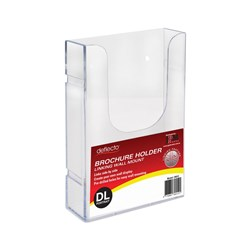Jaybel Office Choice
\$154.50 \$169.95 inc GST ex GST (EACH)
Increase value Decrease value
\$27.23 \$29.95 inc GST ex GST (EACH)
Increase value Decrease value
\$14.50 \$15.95 inc GST ex GST (EACH)
Increase value Decrease value\$7.28 \$8.00 inc GST ex GST (EACH)
Increase value Decrease value
\$12.32 \$13.55 inc GST ex GST (EACH)
Increase value Decrease value
\$7.95 \$8.74 inc GST ex GST (EACH)
Increase value Decrease value
\$5.86 \$6.44 inc GST ex GST (EACH)
Increase value Decrease value
\$57.23 \$62.95 inc GST ex GST (EACH)
Increase value Decrease value
\$5.36 \$5.89 inc GST ex GST (EACH)
Increase value Decrease value
\$2.68 \$2.94 inc GST ex GST (EACH)
Increase value Decrease value
\$28.14 \$30.95 inc GST ex GST (EACH)
Increase value Decrease value
\$10.59 \$11.64 inc GST ex GST (EACH)
Increase value Decrease value